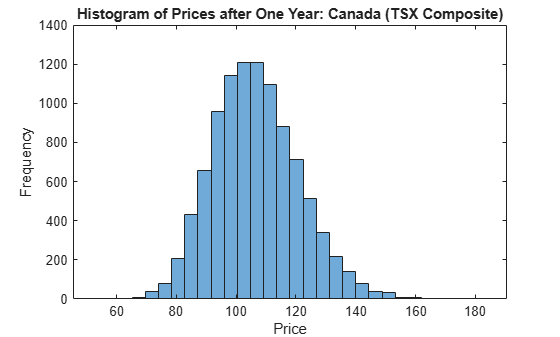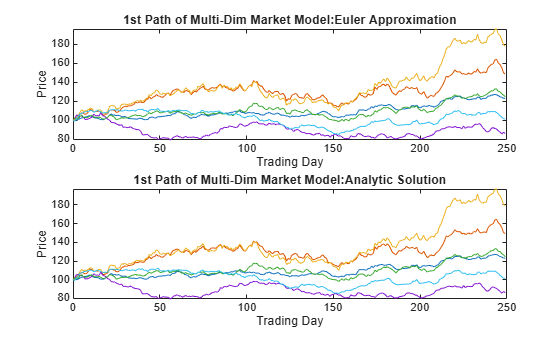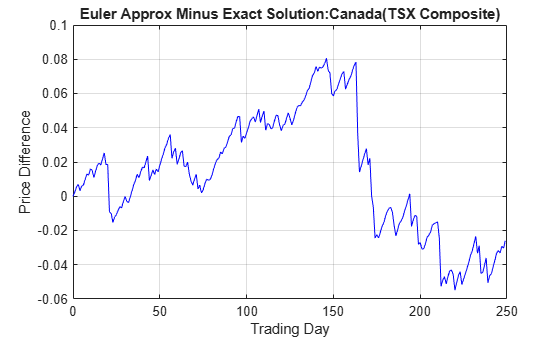# simBySolution

Simulate approximate solution of diagonal-drift `GBM` processes

## Syntax

``[Paths,Times,Z] = simBySolution(MDL,NPeriods)``
``[Paths,Times,Z] = simBySolution(___,Name,Value)``

## Description

example

````[Paths,Times,Z] = simBySolution(MDL,NPeriods)` simulates approximate solution of diagonal-drift for geometric Brownian motion (GBM) processes.```

example

````[Paths,Times,Z] = simBySolution(___,Name,Value)` adds optional name-value pair arguments. You can perform quasi-Monte Carlo simulations using the name-value arguments for `MonteCarloMethod`, `QuasiSequence` and `BrownianMotionMethod`. For more information, see Quasi-Monte Carlo Simulation.```

## Examples

collapse all

Use GBM simulation functions. Separable GBM models have two specific simulation functions:

• An overloaded Euler simulation function (`simulate`), designed for optimal performance.

• A `simBySolution` function that provides an approximate solution of the underlying stochastic differential equation, designed for accuracy.

Load the `Data_GlobalIdx2` data set and specify the SDE model as in Representing Market Models Using SDE Objects, and the GBM model as in Representing Market Models Using SDELD, CEV, and GBM Objects.

```load Data_GlobalIdx2 prices = [Dataset.TSX Dataset.CAC Dataset.DAX ... Dataset.NIK Dataset.FTSE Dataset.SP]; returns = tick2ret(prices); nVariables = size(returns,2); expReturn = mean(returns); sigma = std(returns); correlation = corrcoef(returns); t = 0; X = 100; X = X(ones(nVariables,1)); F = @(t,X) diag(expReturn)* X; G = @(t,X) diag(X) * diag(sigma); SDE = sde(F, G, 'Correlation', ... correlation, 'StartState', X); GBM = gbm(diag(expReturn),diag(sigma), 'Correlation', ... correlation, 'StartState', X);```

To illustrate the performance benefit of the overloaded Euler approximation function (`simulate`), increase the number of trials to `10000`.

```nPeriods = 249; % # of simulated observations dt = 1; % time increment = 1 day rng(142857,'twister') [X,T] = simulate(GBM, nPeriods, 'DeltaTime', dt, ... 'nTrials', 10000); whos X```
``` Name Size Bytes Class Attributes X 250x6x10000 120000000 double ```

Using this sample size, examine the terminal distribution of Canada's TSX Composite to verify qualitatively the lognormal character of the data.

```histogram(squeeze(X(end,1,:)), 30), xlabel('Price'), ylabel('Frequency') title('Histogram of Prices after One Year: Canada (TSX Composite)')```Simulate 10 trials of the solution and plot the first trial:

```rng('default') [S,T] = simulate(SDE, nPeriods, 'DeltaTime', dt, 'nTrials', 10); rng('default') [X,T] = simBySolution(GBM, nPeriods,... 'DeltaTime', dt, 'nTrials', 10); subplot(2,1,1) plot(T, S(:,:,1)), xlabel('Trading Day'),ylabel('Price') title('1st Path of Multi-Dim Market Model:Euler Approximation') subplot(2,1,2) plot(T, X(:,:,1)), xlabel('Trading Day'),ylabel('Price') title('1st Path of Multi-Dim Market Model:Analytic Solution')```In this example, all parameters are constants, and `simBySolution` does indeed sample the exact solution. The details of a single index for any given trial show that the price paths of the Euler approximation and the exact solution are close, but not identical.

The following plot illustrates the difference between the two functions:

```subplot(1,1,1) plot(T, S(:,1,1) - X(:,1,1), 'blue'), grid('on') xlabel('Trading Day'), ylabel('Price Difference') title('Euler Approx Minus Exact Solution:Canada(TSX Composite)')```The `simByEuler` Euler approximation literally evaluates the stochastic differential equation directly from the equation of motion, for some suitable value of the `dt` time increment. This simple approximation suffers from discretization error. This error can be attributed to the discrepancy between the choice of the dt time increment and what in theory is a continuous-time parameter.

The discrete-time approximation improves as `DeltaTime` approaches zero. The Euler function is often the least accurate and most general method available. All models shipped in the simulation suite have the `simByEuler` function.

In contrast, the `simBySolution` function provides a more accurate description of the underlying model. This function simulates the price paths by an approximation of the closed-form solution of separable models. Specifically, it applies a Euler approach to a transformed process, which in general is not the exact solution to this `GBM` model. This is because the probability distributions of the simulated and true state vectors are identical only for piecewise constant parameters.

When all model parameters are piecewise constant over each observation period, the simulated process is exact for the observation times at which the state vector is sampled. Since all parameters are constants in this example, `simBySolution` does indeed sample the exact solution.

For an example of how to use `simBySolution` to optimize the accuracy of solutions, see Optimizing Accuracy: About Solution Precision and Error.

This example shows how to use `simBySolution` with a GBM model to perform a quasi-Monte Carlo simulation. Quasi-Monte Carlo simulation is a Monte Carlo simulation that uses quasi-random sequences instead pseudo random numbers.

Load the `Data_GlobalIdx2` data set and specify the GBM model as in Representing Market Models Using SDELD, CEV, and GBM Objects.

```load Data_GlobalIdx2 prices = [Dataset.TSX Dataset.CAC Dataset.DAX ... Dataset.NIK Dataset.FTSE Dataset.SP]; returns = tick2ret(prices); nVariables = size(returns,2); expReturn = mean(returns); sigma = std(returns); correlation = corrcoef(returns); X = 100; X = X(ones(nVariables,1)); GBM = gbm(diag(expReturn),diag(sigma), 'Correlation', ... correlation, 'StartState', X);```

Perform a quasi-Monte Carlo simulation by using `simBySolution` with the optional name-value arguments for `'MonteCarloMethod'`,`'QuasiSequence'`, and `'BrownianMotionMethod'`.

`[paths,time,z] = simBySolution(GBM, 10,'ntrials',4096,'MonteCarloMethod','quasi','QuasiSequence','sobol','BrownianMotionMethod','brownian-bridge');`

## Input Arguments

collapse all

Geometric Brownian motion (GBM) model, specified as a `gbm` object that is created using `gbm`.

Data Types: `object`

Number of simulation periods, specified as a positive scalar integer. The value of `NPeriods` determines the number of rows of the simulated output series.

Data Types: `double`

### Name-Value Arguments

Specify optional pairs of arguments as `Name1=Value1,...,NameN=ValueN`, where `Name` is the argument name and `Value` is the corresponding value. Name-value arguments must appear after other arguments, but the order of the pairs does not matter.

Before R2021a, use commas to separate each name and value, and enclose `Name` in quotes.

Example: ```[Paths,Times,Z] = simBySolution(GBM,NPeriods,'DeltaTime',dt,'NTrials',10)```

Simulated trials (sample paths) of `NPERIODS` observations each, specified as the comma-separated pair consisting of `'NTrials'` and a positive scalar integer.

Data Types: `double`

Positive time increments between observations, specified as the comma-separated pair consisting of `'DeltaTime'` and a scalar or a `NPERIODS`-by-`1` column vector.

`DeltaTime` represents the familiar dt found in stochastic differential equations, and determines the times at which the simulated paths of the output state variables are reported.

Data Types: `double`

Number of intermediate time steps within each time increment dt (specified as `DeltaTime`), specified as the comma-separated pair consisting of `'NSteps'` and a positive scalar integer.

The `simBySolution` function partitions each time increment dt into `NSteps` subintervals of length dt/`NSteps`, and refines the simulation by evaluating the simulated state vector at `NSteps − 1` intermediate points. Although `simBySolution` does not report the output state vector at these intermediate points, the refinement improves accuracy by allowing the simulation to more closely approximate the underlying continuous-time process.

Data Types: `double`

Flag to indicate whether `simBySolution` uses antithetic sampling to generate the Gaussian random variates that drive the Brownian motion vector (Wiener processes), specified as the comma-separated pair consisting of `'Antithetic'` and a scalar logical flag with a value of `True` or `False`.

When you specify `True`, `simBySolution` performs sampling such that all primary and antithetic paths are simulated and stored in successive matching pairs:

• Odd trials `(1,3,5,...)` correspond to the primary Gaussian paths.

• Even trials `(2,4,6,...)` are the matching antithetic paths of each pair derived by negating the Gaussian draws of the corresponding primary (odd) trial.

Note

If you specify an input noise process (see `Z`), `simBySolution` ignores the value of `Antithetic`.

Data Types: `logical`

Monte Carlo method to simulate stochastic processes, specified as the comma-separated pair consisting of `'MonteCarloMethod'` and a string or character vector with one of the following values:

• `"standard"` — Monte Carlo using pseudo random numbers

• `"quasi"` — Quasi-Monte Carlo using low-discrepancy sequences

• `"randomized-quasi"` — Randomized quasi-Monte Carlo

Note

If you specify an input noise process (see `Z`), `simBySolution` ignores the value of `MonteCarloMethod`.

Data Types: `string` | `char`

Low discrepancy sequence to drive the stochastic processes, specified as the comma-separated pair consisting of `'QuasiSequence'` and a string or character vector with the following value:

• `"sobol"` — Quasi-random low-discrepancy sequences that use a base of two to form successively finer uniform partitions of the unit interval and then reorder the coordinates in each dimension

Note

• If `MonteCarloMethod` option is not specified or specified as`"standard"`, `QuasiSequence` is ignored.

• If you specify an input noise process (see `Z`), `simBySolution` ignores the value of `QuasiSequence`.

Data Types: `string` | `char`

Brownian motion construction method, specified as the comma-separated pair consisting of `'BrownianMotionMethod'` and a string or character vector with one of the following values:

• `"standard"` — The Brownian motion path is found by taking the cumulative sum of the Gaussian variates.

• `"brownian-bridge"` — The last step of the Brownian motion path is calculated first, followed by any order between steps until all steps have been determined.

• `"principal-components"` — The Brownian motion path is calculated by minimizing the approximation error.

Note

If an input noise process is specified using the `Z` input argument, `BrownianMotionMethod` is ignored.

The starting point for a Monte Carlo simulation is the construction of a Brownian motion sample path (or Wiener path). Such paths are built from a set of independent Gaussian variates, using either standard discretization, Brownian-bridge construction, or principal components construction.

Both standard discretization and Brownian-bridge construction share the same variance and, therefore, the same resulting convergence when used with the `MonteCarloMethod` using pseudo random numbers. However, the performance differs between the two when the `MonteCarloMethod` option `"quasi"` is introduced, with faster convergence for the `"brownian-bridge"` construction option and the fastest convergence for the `"principal-components"` construction option.

Data Types: `string` | `char`

Direct specification of the dependent random noise process used to generate the Brownian motion vector (Wiener process) that drives the simulation, specified as the comma-separated pair consisting of `'Z'` and a function or as an ```(NPERIODS * NSTEPS)```-by-`NBROWNS`-by-`NTRIALS` three-dimensional array of dependent random variates.

The input argument `Z` allows you to directly specify the noise generation process. This process takes precedence over the `Correlation` parameter of the input `gbm` object and the value of the `Antithetic` input flag.

Note

If you specify `Z` as a function, it must return an `NBROWNS`-by-`1` column vector, and you must call it with two inputs:

• A real-valued scalar observation time t.

• An `NVARS`-by-`1` state vector Xt.

Data Types: `double` | `function`

Flag that indicates how the output array `Paths` is stored and returned, specified as the comma-separated pair consisting of `'StorePaths'` and a scalar logical flag with a value of `True` or `False`.

• If `StorePaths` is `True` (the default value) or is unspecified, `simBySolution` returns `Paths` as a three-dimensional time series array.

• If `StorePaths` is `False` (logical `0`), `simBySolution` returns the `Paths` output array as an empty matrix.

Data Types: `logical`

Sequence of end-of-period processes or state vector adjustments, specified as the comma-separated pair consisting of `'Processes'` and a function or cell array of functions of the form

`${X}_{t}=P\left(t,{X}_{t}\right)$`

The `simBySolution` function runs processing functions at each interpolation time. They must accept the current interpolation time t, and the current state vector Xt, and return a state vector that may be an adjustment to the input state.

`simBySolution` applies processing functions at the end of each observation period. These functions must accept the current observation time t and the current state vector Xt, and return a state vector that may be an adjustment to the input state.

The end-of-period `Processes` argument allows you to terminate a given trial early. At the end of each time step, `simBySolution` tests the state vector Xt for an all-`NaN` condition. Thus, to signal an early termination of a given trial, all elements of the state vector Xt must be `NaN`. This test enables a user-defined `Processes` function to signal early termination of a trial, and offers significant performance benefits in some situations (for example, pricing down-and-out barrier options).

If you specify more than one processing function, `simBySolution` invokes the functions in the order in which they appear in the cell array. You can use this argument to specify boundary conditions, prevent negative prices, accumulate statistics, plot graphs, and more.

Data Types: `cell` | `function`

## Output Arguments

collapse all

Simulated paths of correlated state variables, returned as an ```(NPERIODS + 1)```-by-`NVARS`-by-`NTRIALS` three-dimensional time series array.

For a given trial, each row of `Paths` is the transpose of the state vector Xt at time t. When the input flag `StorePaths` = `False`, `simBySolution` returns `Paths` as an empty matrix.

Observation times associated with the simulated paths, returned as an `(NPERIODS + 1)`-by-`1` column vector. Each element of `Times` is associated with the corresponding row of `Paths`.

Dependent random variates used to generate the Brownian motion vector (Wiener processes) that drive the simulation, returned as an ```(NPERIODS * NSTEPS)```-by-`NBROWNS`-by-`NTRIALS` three-dimensional time series array.

collapse all

### Antithetic Sampling

Simulation methods allow you to specify a popular variance reduction technique called antithetic sampling.

This technique attempts to replace one sequence of random observations with another of the same expected value, but smaller variance. In a typical Monte Carlo simulation, each sample path is independent and represents an independent trial. However, antithetic sampling generates sample paths in pairs. The first path of the pair is referred to as the primary path, and the second as the antithetic path. Any given pair is independent of any other pair, but the two paths within each pair are highly correlated. Antithetic sampling literature often recommends averaging the discounted payoffs of each pair, effectively halving the number of Monte Carlo trials.

This technique attempts to reduce variance by inducing negative dependence between paired input samples, ideally resulting in negative dependence between paired output samples. The greater the extent of negative dependence, the more effective antithetic sampling is.

## Algorithms

The `simBySolution` function simulates `NTRIALS` sample paths of `NVARS` correlated state variables, driven by `NBROWNS` Brownian motion sources of risk over `NPERIODS` consecutive observation periods, approximating continuous-time GBM short-rate models by an approximation of the closed-form solution.

Consider a separable, vector-valued GBM model of the form:

`$d{X}_{t}=\mu \left(t\right){X}_{t}dt+D\left(t,{X}_{t}\right)V\left(t\right)d{W}_{t}$`

where:

• Xt is an `NVARS`-by-`1` state vector of process variables.

• μ is an `NVARS`-by-`NVARS` generalized expected instantaneous rate of return matrix.

• V is an `NVARS`-by-`NBROWNS` instantaneous volatility rate matrix.

• dWt is an `NBROWNS`-by-`1` Brownian motion vector.

The `simBySolution` function simulates the state vector Xt using an approximation of the closed-form solution of diagonal-drift models.

When evaluating the expressions, `simBySolution` assumes that all model parameters are piecewise-constant over each simulation period.

In general, this is not the exact solution to the models, because the probability distributions of the simulated and true state vectors are identical only for piecewise-constant parameters.

When parameters are piecewise-constant over each observation period, the simulated process is exact for the observation times at which Xt is sampled.

Gaussian diffusion models, such as `hwv`, allow negative states. By default, `simBySolution` does nothing to prevent negative states, nor does it guarantee that the model be strictly mean-reverting. Thus, the model may exhibit erratic or explosive growth.

 Aït-Sahalia, Yacine. “Testing Continuous-Time Models of the Spot Interest Rate.” Review of Financial Studies, Vol. 9, No. 2 ( Apr. 1996): 385–426.

 Aït-Sahalia, Yacine. “Transition Densities for Interest Rate and Other Nonlinear Diffusions.” The Journal of Finance, Vol. 54, No. 4 (Aug. 1999): 1361–95.

 Glasserman, Paul. Monte Carlo Methods in Financial Engineering, New York: Springer-Verlag, 2004.

 Hull, John C. Options, Futures and Other Derivatives, 7th ed, Prentice Hall, 2009.

 Johnson, Norman Lloyd, Samuel Kotz, and Narayanaswamy Balakrishnan. Continuous Univariate Distributions, 2nd ed. Wiley Series in Probability and Mathematical Statistics. New York: Wiley, 1995.

 Shreve, Steven E. Stochastic Calculus for Finance, New York: Springer-Verlag, 2004.